# Cutting Setting Triangles

After languishing on the design wall for over a month, the Mediterranean Rose quilt has finally made it onto the longarm frame. I have high hopes for getting it quilted this week, though I need the design wall more!

This quilt has blocks set on point, which requires corner and side triangles to complete the square shape. So let's have a refresher course on measuring and cutting side and corner triangles for quilts with those on-point layouts, no matter if the finished quilt is square or rectangular.

In the picture below, the Side Triangles are in black (for this layout there are 2), and the Corner Triangles are in red (there are 4).

## Side Triangles

The process below makes 4 (four) side triangles. Repeat the steps for more side triangles, or follow the Corner Triangle process to make just 2 (two).

To figure what size square to cut for Side Triangles, measure the short side of the quilt's side triangle area (in the blue oval), or use the finished block size of the quilt blocks. Personally, I like to measure, because fabric has a way of either growing or shrinking!

Multiply that measurement by 1.414, then add 1 1/4" (or 1.25). Round up to the nearest 1/8" (or add .125).

Side Triangle Formula:
(side measurement x 1.414) + 1.25; round up to nearest 1/8 (or add .125).

Example: (10" x 1.414) + 1.25 = 15.39. Rounded up, I'd cut the square at 15 1/2" (15.50).

The next step is to cut the square on both diagonals, yielding 4 side setting triangles. I love this because it creates the straight of grain on the outside edge for the quilt.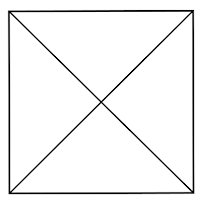straight of grain on outer edge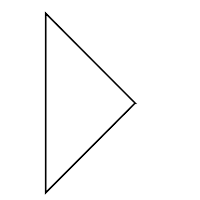Makes 4 (four) side triangles

### Corner Triangles

The process below makes 2 (two). Repeat the steps to make a total of 4 (four) for all the corners.

To figure what size square to cut for the Corner Triangles, measure the long edge of the quilt top corner, as circled in blue above.

Multiply that measurement by .707 and add 7/8 (or .875), and round up to the nearest 1/8" (or add .125) for the size square to cut.

Corner Triangle formula:
(corner measurement x .707) + .875; round to the nearest 1/8 (or add .125).

This time, cut the square on one diagonal, making a half square triangle unit.straight of grain on outer edges of corners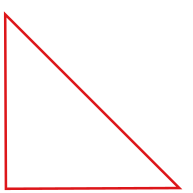Makes 2 (two) Corner Triangles

And there you have it - the math to make any size triangle for an on-point quilt.

Enjoy!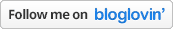Need more quilting tutorials? Check out the Tutorial page!

1.You are a genius! Thanks for sharing this mysterious information....

2.Such a gorgeous quilt - can't wait to see it finished! Thank you so much for the triangle formulas. It's so timely - just so happens I need to cut some!

3.4.Susan this quilt is beautiful. Is it your pattern? Are you on Bluprint?

5.I love how blocks look on point. Thanks for the lesson on the setting triangles. I would have never figured this out, so I'm saving this.

6.That is a stunning quilt. Thanks for the tutorial on setting triangles.

7.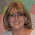In our beginner quilting class, we have the students measure the length of the outside edge where the setting triangle will go (along the black line that runs vertical, from top point to bottom point). Add an inch and that is the size they cut their square. The rest of the process is the same as yours so the bias edges are on the inside. It's easy for them, and they don't hate me for giving them another math formula. :) (Formula's for HSTs drive them nuts…) In our next class I'm going to give your formula a try. Thanks so much for sharing!

8.Such a beautiful quilt! Thank you for sharing the formulas for calculating those setting triangles. By any chance, do you have a formula for calculating the width of a block on point? I'd like to try placing some blocks on point but don't know how to calculate how wide my quilt would be. I don't know how you figured out to multiply by 1.414!

1.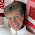Aw, thanks Patti, but really, it's geometry to thank for the 1.414 multiplier.

The diagonal (or point to point width) of a block, is the formula:
x times the square root of 2, with x being the length of one edge of the square
And 1.414 just happens to be the square root of 2!

So, to find out that diagonal block width, multiply the edge length by 1.414, then multiply the result by how many blocks go across or down the quilt to figure it's size.
ex: 8" block x 1.414 = 11.3" 11.3" x 5 blocks across = 56.56"

9.Beautiful quilt Susan and a great lesson on figuring block sizes!

10.I have a handout someone gave me years ago with all the math done. I love my cheat sheet!!

Thank you so much for commenting...you just made my day!